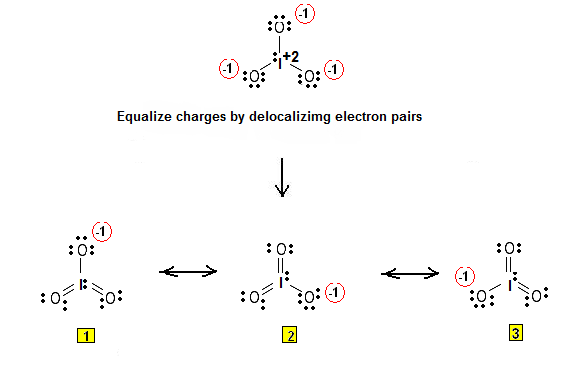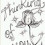Simple Procedure for writing Lewis Structures – Lewis Structures for iodate ion IO3- | Chemistry Net

# Simple Procedure for writing Lewis Structures – Lewis Structures for iodate ion IO3-

A simple procedure for writing Lewis structures is given in a previous article entitled “Lewis Structures and the Octet Rule”. Relevant worked examples were given in the following articles: Examples #1, #2, #3 and #4, #5, #6 and #7.

Another example  for writing Lewis structures following the above procedure is given bellow:

Let us consider the case of  IO3- :

Step 1: The central atom will be the I atom since it is the less electronegative. Connect the atoms with single bonds:

Step 2: Calculate the # of electrons in π bonds (multiple bonds) using  formula (1) in the article entitled “Lewis Structures and the Octet Rule”.

:

Where n in this case is 4 since IO3- consists of four atoms.
Where V = (7 + 6 + 6 + 6 ) – (-1) = 26
Therefore, P = 6n + 2 – V = 6 * 4 + 2 – 26 = 0     So,there are no π electrons in IO3-

Step 3 & 4: Therefore, the Lewis structures for IO3-  are as follows:Figure 1: Lewis structures for IO3-. The iodine atom is not restricted to an octet. Double bonds may be generated from the oxygen lone pairs so that to minimize charge separation.

1.1.